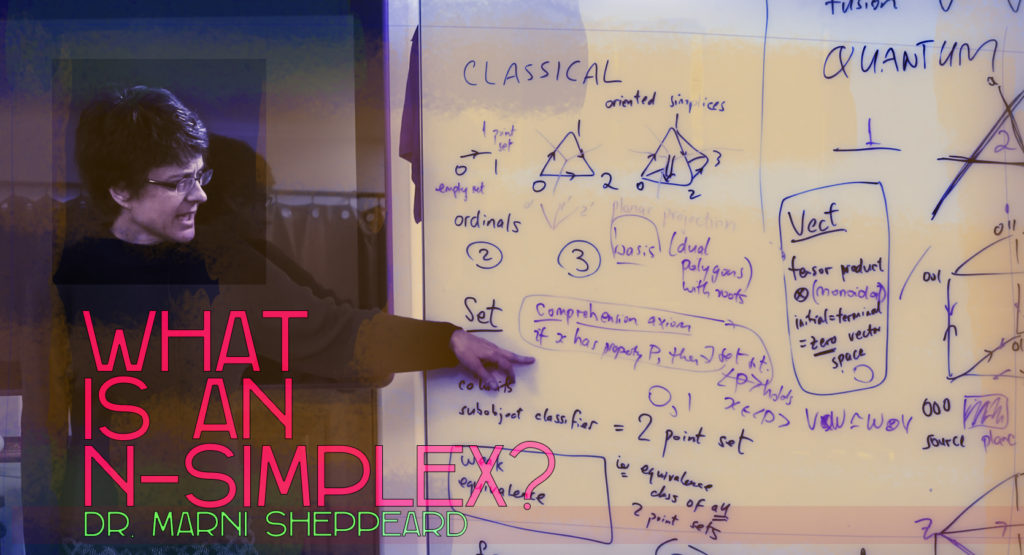Category Theory: What is an n-Simplex?

March 8, 2018BlogDr. Marni Sheppeard talks about Category Theory and n-Simplex

In Quantum Gravity Research‘s (QGR) video ‘Category Theory: What Is An N-Simplex?’,  physicist and QGR researcher Dr. Marni Sheppeard, introduces category theory in the context of   emergence theory and combinatoric connection to the Quasicrystalline Spin Network (QSN). Marni is one of New Zealand’s leading experts on the application of category theory to quantum information.

What is Category Theory?

Category theory is a branch of mathematics which attempts a formalization in terms of simple elements and associations between them.

The simplest building block in geometry is always the simplex.

What is an n-simplex? The nsimplex is the smallest figure that contains n + 1 given points in ndimensional space and that does not lie in a space of lower dimension. Simplex-integers are the most powerful numbers to express counting function and set theoretic information.

If you’re familiar with QGR’s work, you may have discovered that emergence theory recognizes quasicrystals as maximally efficient codes. And an n-dimensional quasicrystal is a network of quasicrystals in all dimensions lower than it.

Quasicrystals are the most efficient codes possible in the universe of all codes.

Category theory and the n-simplex in the context of emergence theory is important.  We want the combinatorics and geometry of basic simplices and cubes to fit into the context of topos theory and category theory, so that we can do quantum logic using canonical axioms. Classical simplices count numbers with cardinalities, while vector space simplices (for quantum mechanics) naturally use integral dimensions.

Want to know more about the QSN? Here’s a little Visualizing a Hypothetical Planck Scale Substructure of Reality featuring geometer, animator and QGR research scientist Dugan Hammock displaying some of his work visualizing the quasicrystalline point space on which we model our physics.

Learn more about special dimensions related to maximally efficient networks, “code power” and two letter codes,  quasicrystal code possibility spaces, the non-locality of crystals, and touch upon other aspects of the conceptual framework of emergence theory, an approach to unification physics based on the quasicrystalline spin network in the paper ‘Toward the Unification of Physics and Number Theory’.

Want to see more Quantum Gravity Research videos? Subscribe to our Quantum Gravity Research Youtube Channel

Save

Save

Save

Save

Save

Save

Save

Save

Save

Save

Save

Save

Save

Save

Save

Save

Save